# NCERT Solutions For Class 10 Maths Chapter 5 Exercise 5.1- Arithmetic Progressions

Download NCERT Solutions For Class 10 Maths Chapter 5 Exercise 5.1 – Arithmetic Progressions This Exercise contains 4 questions, for which detailed answers have been provided in this note. In case you are looking at studying the remaining Exercise for Class 10 for Maths NCERT solutions for other Chapters, you can click the link at the end of this Note.

### NCERT Solutions For Class 10 Maths Chapter 5 Exercise 5.1- Arithmetic Progressions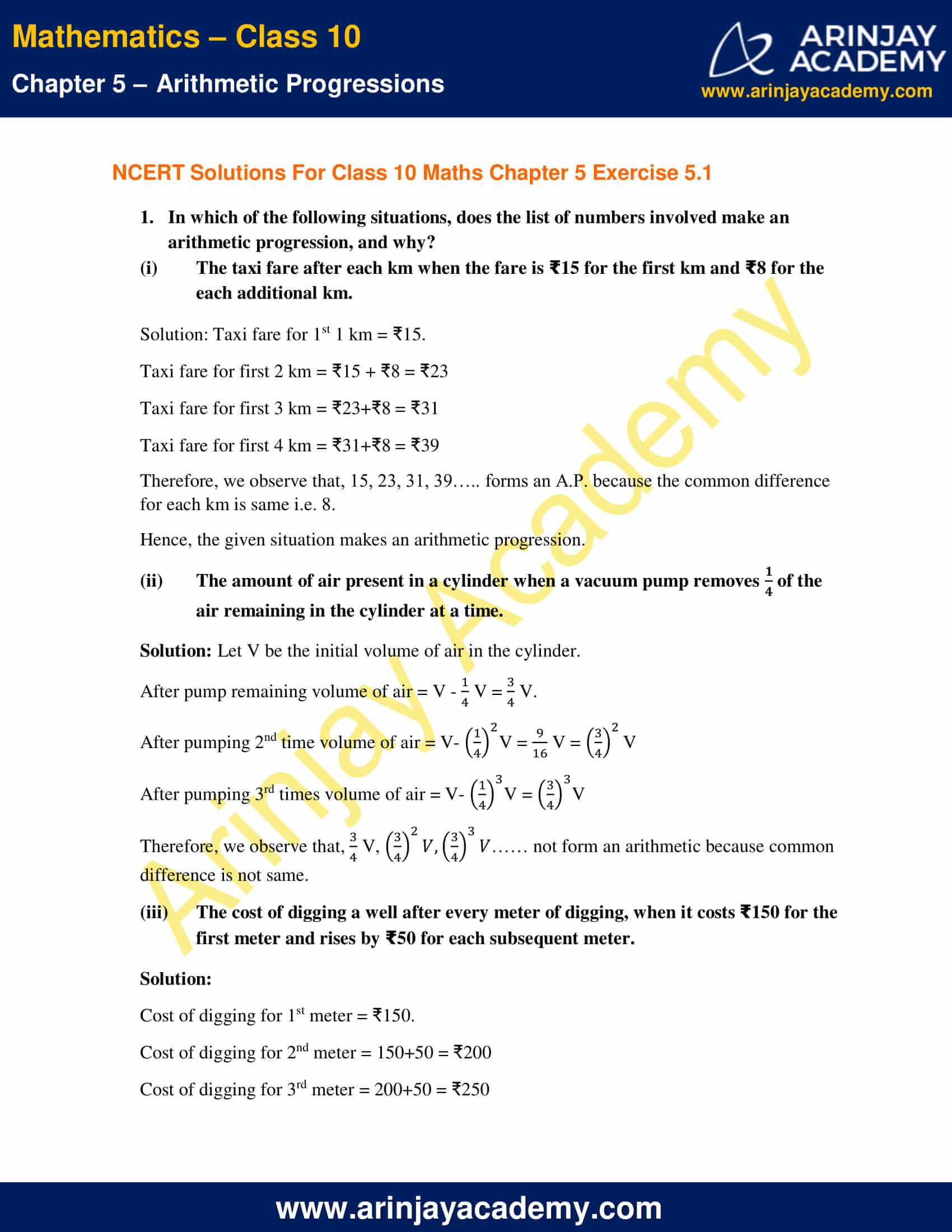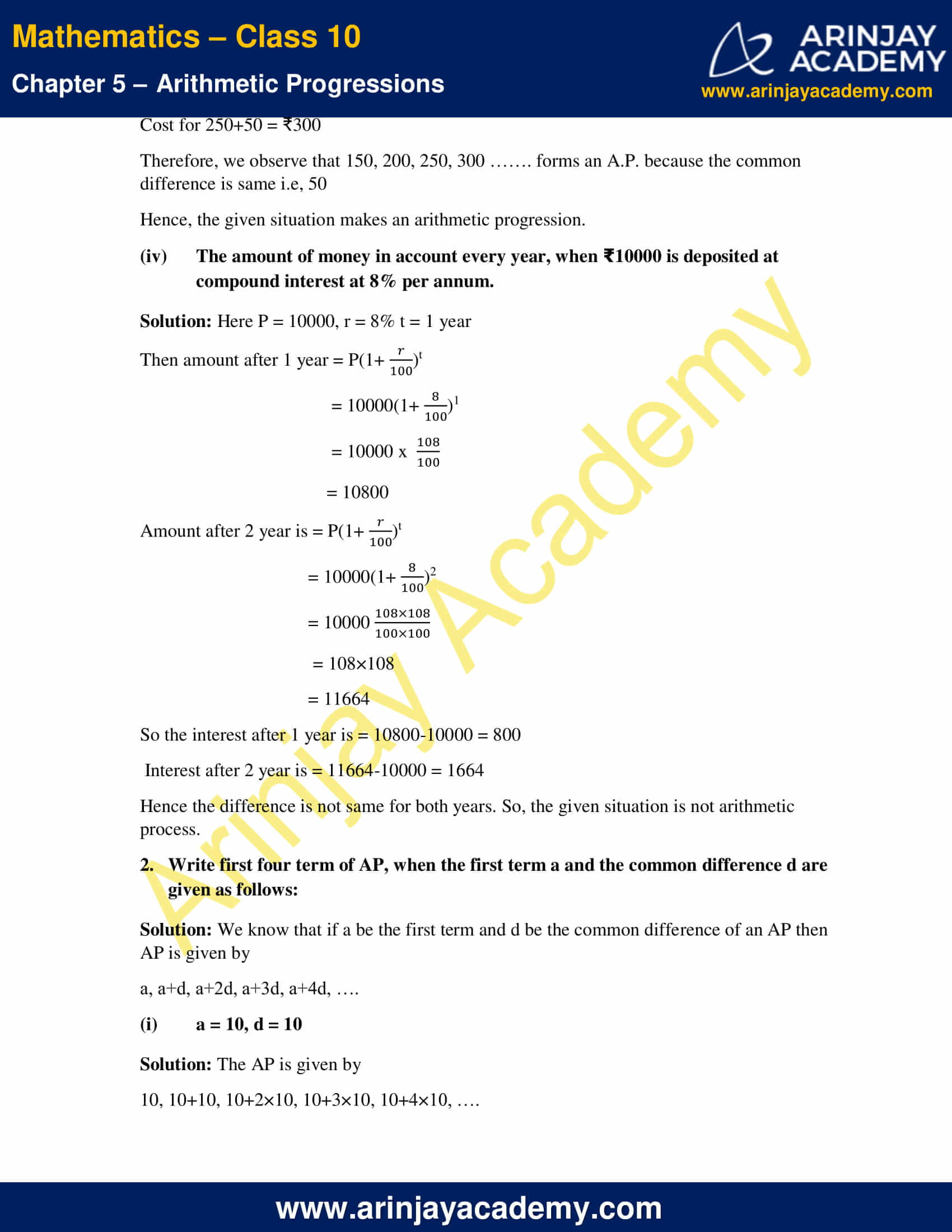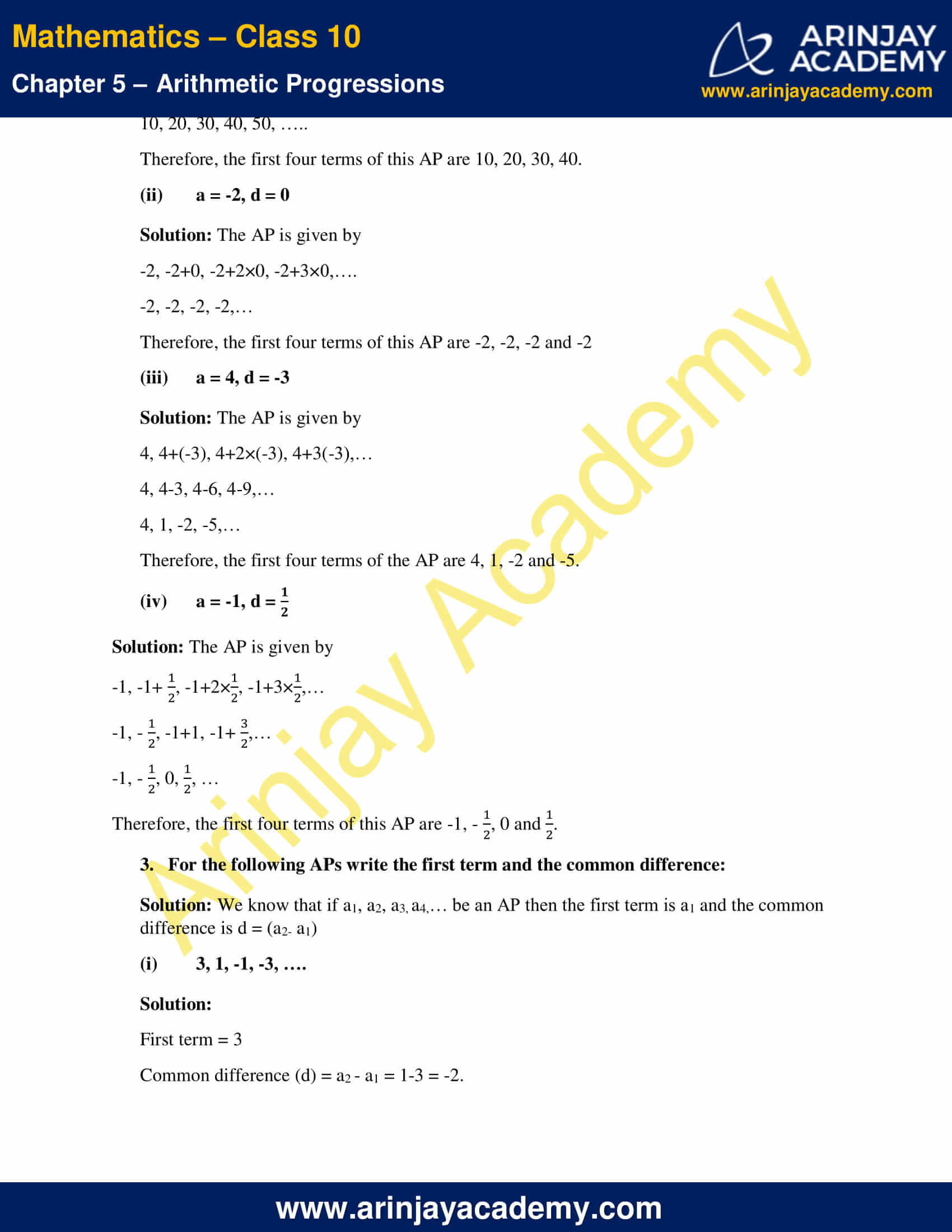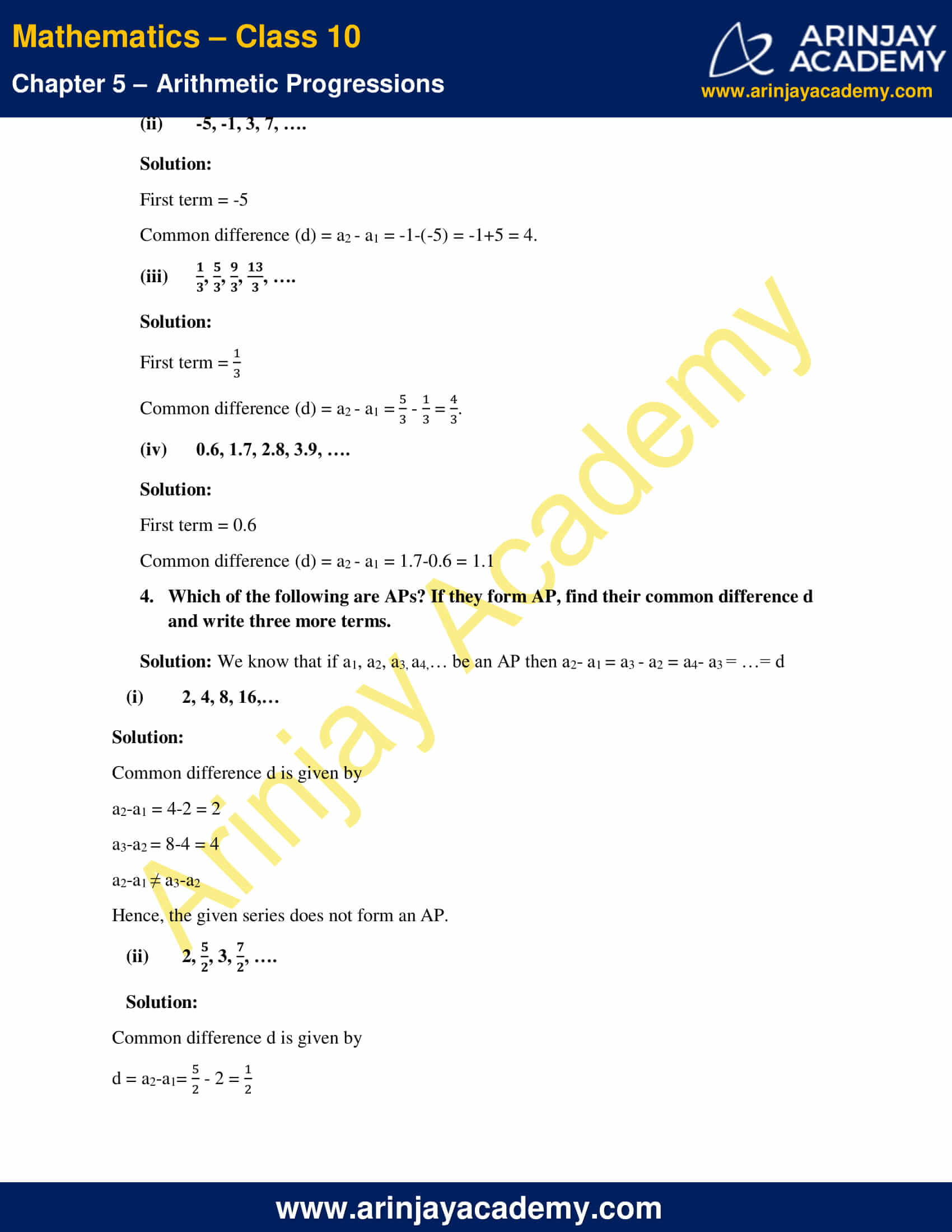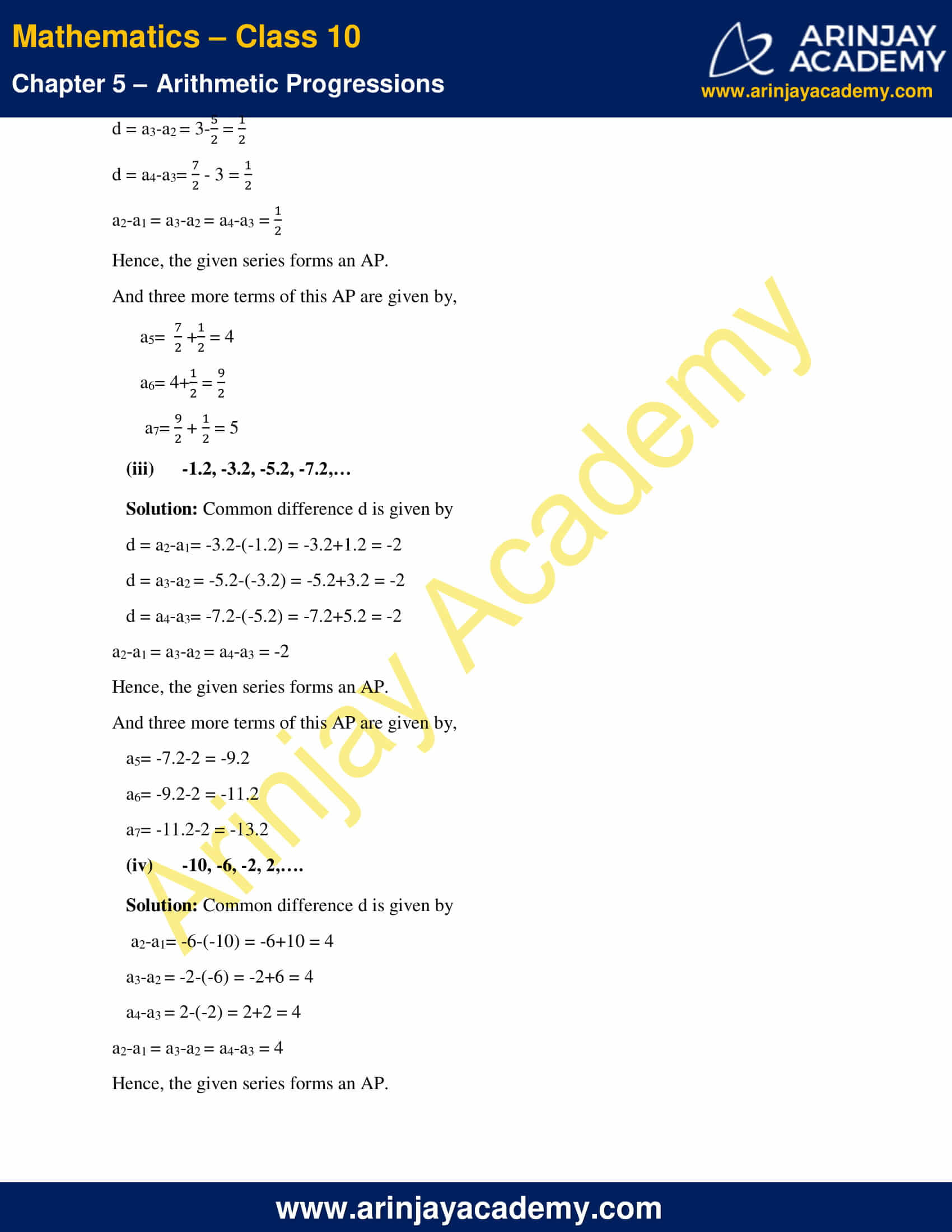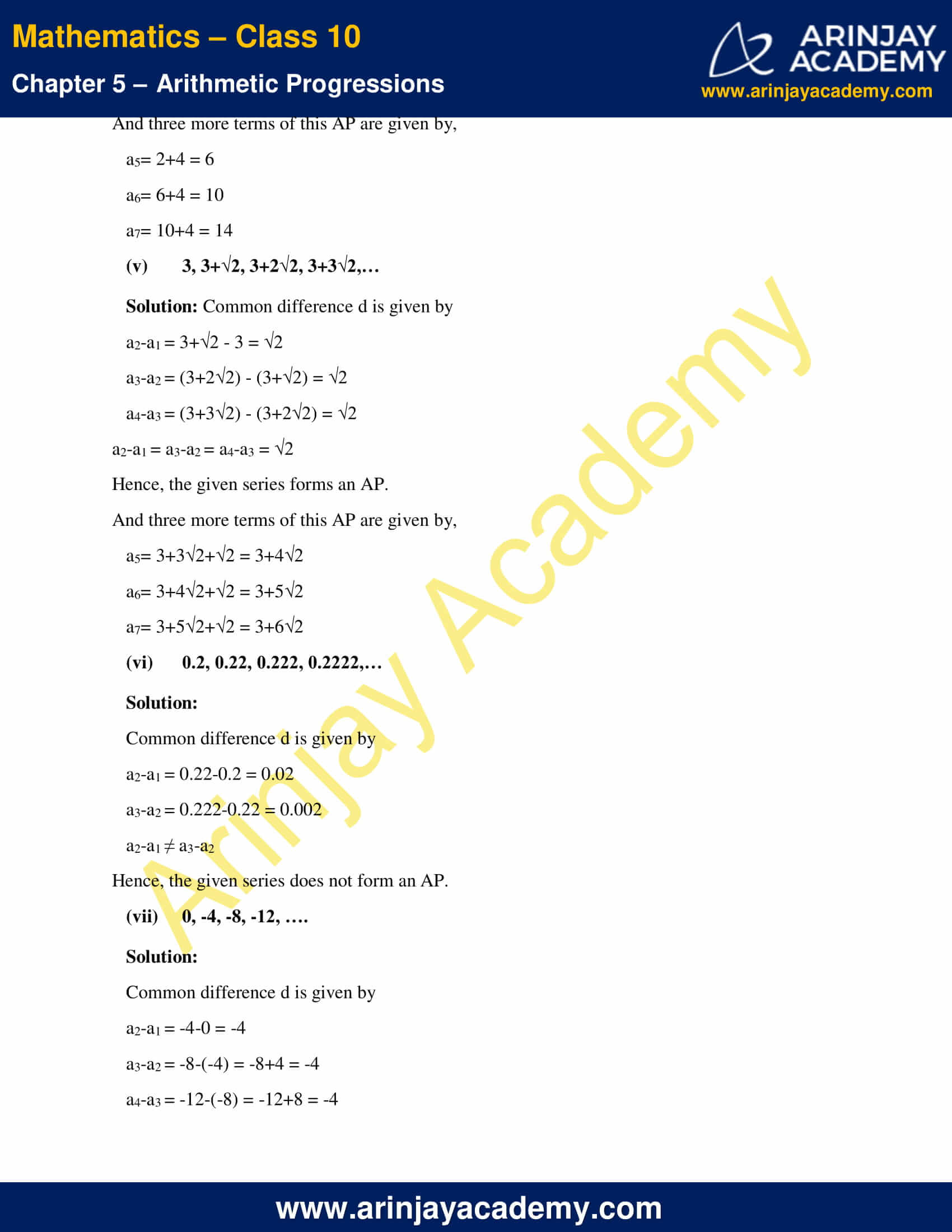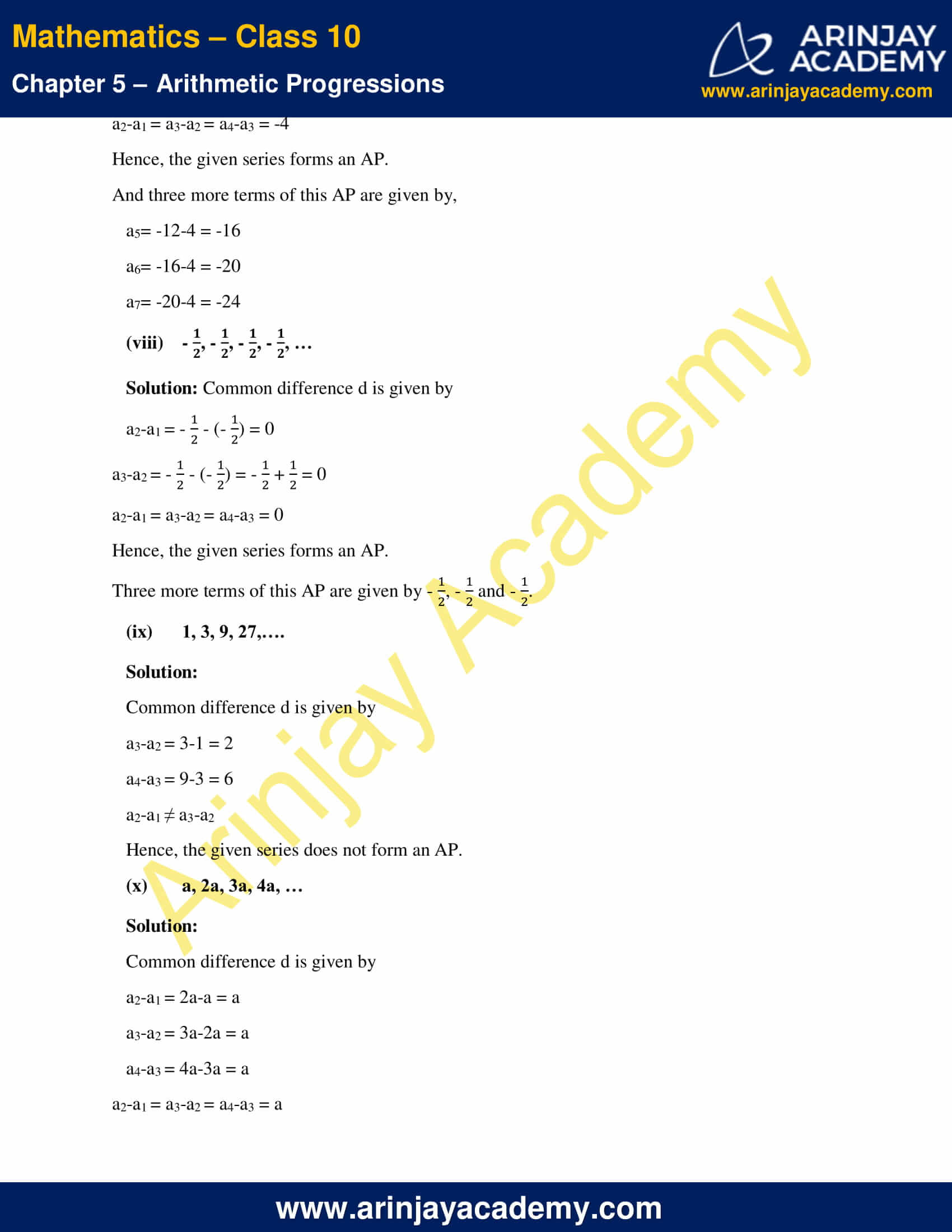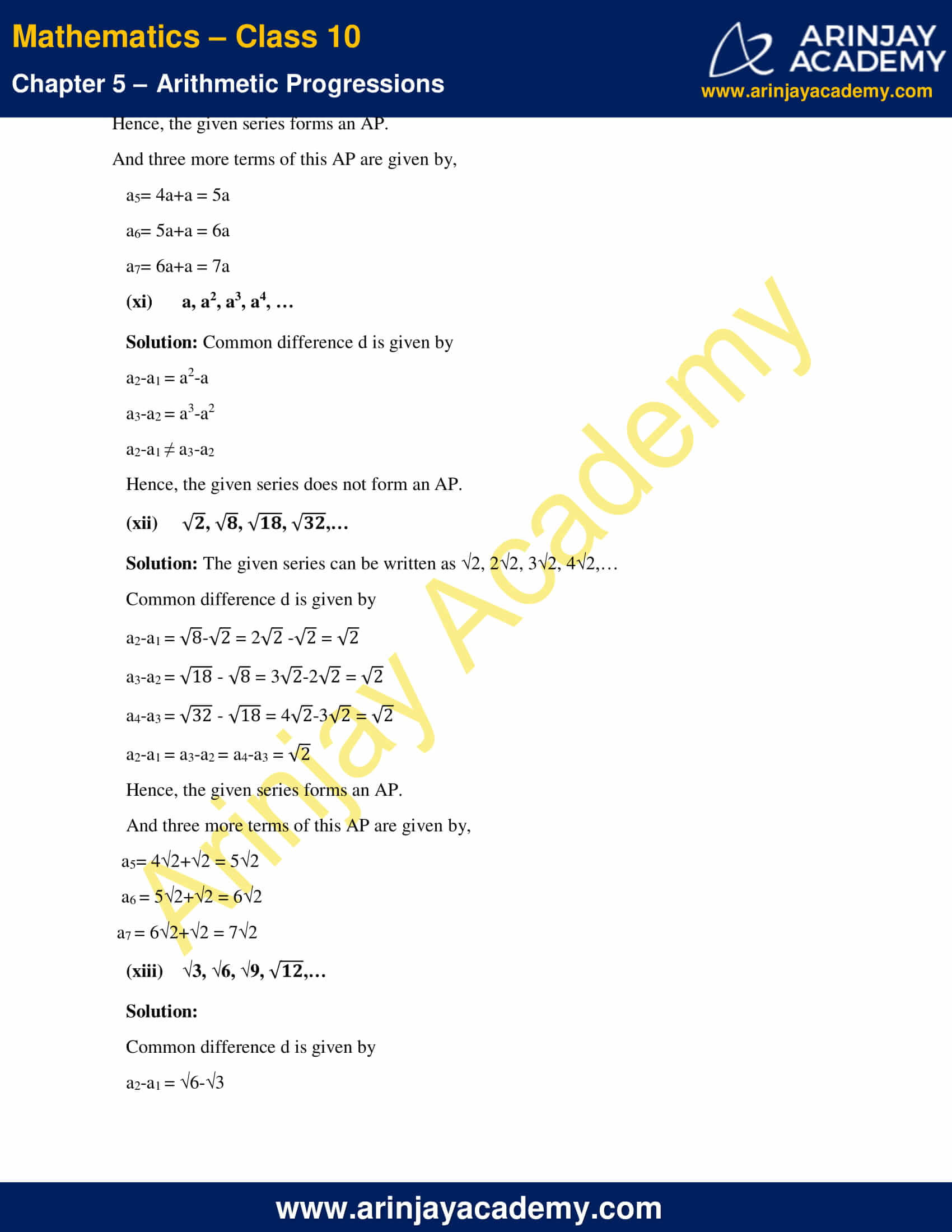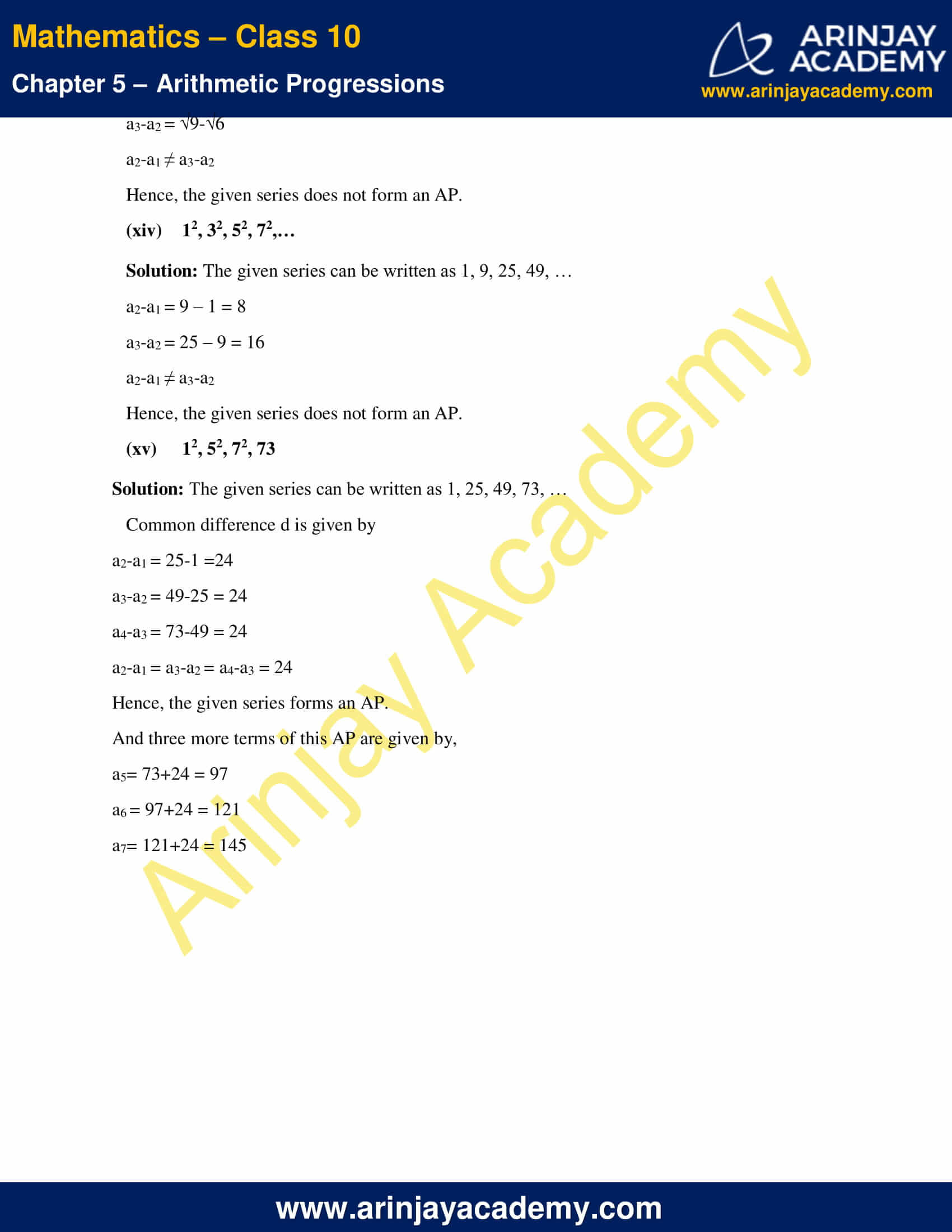NCERT Solutions For Class 10 Maths Chapter 5 Exercise 5.1- Arithmetic Progressions

1. In which of the following situations, does the list of numbers involved make an arithmetic progression, and why?

(i) The taxi fare after each km when the fare is 15 for the first km and 8 for the each additional km.

Solution: Taxi fare for 1st 1 km = ₹15.
Taxi fare for first 2 km = ₹15 + ₹8 = ₹23
Taxi fare for first 3 km = ₹23+₹8 = ₹31
Taxi fare for first 4 km = ₹31+₹8 = ₹39
Therefore, we observe that, 15, 23, 31, 39….. forms an A.P. because the common difference for each km is same i.e. 8.
Hence, the given situation makes an arithmetic progression.

(ii) The amount of air present in a cylinder when a vacuum pump removes (1/4) of the air remaining in the cylinder at a time.

Solution: Let V be the initial volume of air in the cylinder.
After pump remaining volume of air = V – (1/4)V = (3/4)V.
After pumping 2nd time volume of air = V- (1/4)²V = (9/16)V = (3/4)²V
After pumping 3rd times volume of air = V- (1/4)³V = (3/4)³V
Therefore, we observe that,  (3/4)V, (3/4)²V, (3/4)³V …… not form an arithmetic because common difference is not same.

(iii) The cost of digging a well after every meter of digging, when it costs 150 for the first meter and rises by 50 for each subsequent meter.

Solution:

Cost of digging for 1st meter = ₹150.
Cost of digging for 2nd meter = 150+50 = ₹200
Cost of digging for 3rd meter = 200+50 = ₹250
Cost for 250+50 = ₹300
Therefore, we observe that 150, 200, 250, 300 ……. forms an A.P. because the common difference is same i.e, 50
Hence, the given situation makes an arithmetic progression.

(iv) The amount of money in account every year, when 10000 is deposited at compound interest at 8% per annum.

Solution: Here P = 10000, r = 8% t = 1 year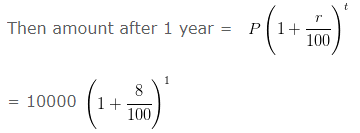= 10000 x (108/100)
= 10800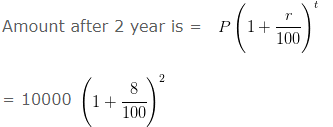= 10000 x (108 x 108/100 x 100)
= 108×108
= 11664
So the interest after 1 year is = 10800-10000 = 800
Interest after 2 year is = 11664-10000 = 1664
Hence the difference is not same for both years. So, the given situation is not arithmetic process.

2. Write first four term of AP, when the first term a and the common difference d are given as follows:

Solution: We know that if a be the first term and d be the common difference of an AP then AP is given by
a, a+d, a+2d, a+3d, a+4d, ….

(i) a = 10, d = 10

Solution: The AP is given by
10, 10+10, 10+2×10, 10+3×10, 10+4×10, ….
10, 20, 30, 40, 50, …..
Therefore, the first four terms of this AP are 10, 20, 30, 40.

(ii) a = -2, d = 0

Solution: The AP is given by
-2, -2+0, -2+2×0, -2+3×0,….
-2, -2, -2, -2,…
Therefore, the first four terms of this AP are -2, -2, -2 and -2

(iii) a = 4, d = -3

Solution: The AP is given by
4, 4+(-3), 4+2×(-3), 4+3(-3),…
4, 4-3, 4-6, 4-9,…
4, 1, -2, -5,…
Therefore, the first four terms of the AP are 4, 1, -2 and -5.

(iv) a = -1, d = 1/2

Solution: The AP is given by
-1, -1+ (1/2), -1+2×(1/2), -1+3×(1/2),…
-1, (-1/2), -1+1, -1+ (3/2),…
-1, (-1/2), 0, (1/2), …
Therefore, the first four terms of this AP are -1, (-1/2), 0 and (1/2).

NCERT solutions for class 10 maths chapter 5 – Question 3. For the following APs write the first term and the common difference:

Solution: We know that if a1, a2, a3, a4,… be an AP then the first term is a1 and the common difference is d = (a2- a1)

(i) 3, 1, -1, -3, ….

Solution:
First term = 3
Common difference (d) = a2 – a1 = 1-3 = -2.

(ii) -5, -1, 3, 7, ….

Solution:
First term = -5
Common difference (d) = a2 – a1 = -1-(-5) = -1+5 = 4.

(iii) 1/3, 5/3, 9/3, 13/3, ….

Solution:

First term = 1/3
Common difference (d) = a2 – a1 = (5/3) – (1/3) = 4/3

(iv) 0.6, 1.7, 2.8, 3.9, ….

Solution:
First term = 0.6
Common difference (d) = a2 – a1 = 1.7-0.6 = 1.1

4. Which of the following are APs? If they form AP, find their common difference d and write three more terms.

Solution: We know that if a1, a2, a3, a4,… be an AP then a2– a1 = a3 – a2 = a4– a3 = …= d

(i) 2, 4, 8, 16,…

Solution:

Common difference d is given by
a2-a1 = 4-2 = 2
a3-a2 = 8-4 = 4
a2-a1 ≠ a3-a2
Hence, the given series does not form an AP.

(ii) 2, 5/2, 3, 7/2, ….

Solution:

Common difference d is given by
d = a2-a1= 5/2 – 2 = 1/2
d = a3-a2 = 3-(5/2) = 1/2
d = a4-a3= (7/2) – 3 = (1/2)
a2-a1 = a3-a2 = a4-a3 = (1/2)
Hence, the given series forms an AP.
And three more terms of this AP are given by,
a5= (7/2) + (1/2) = 4
a6= 4+(1/2) = 9/2
a7= (9/2) + (1/2) = 5

(iii) -1.2, -3.2, -5.2, -7.2,…

Solution: Common difference d is given by
d = a2-a1= -3.2-(-1.2) = -3.2+1.2 = -2
d = a3-a2 = -5.2-(-3.2) = -5.2+3.2 = -2
d = a4-a3= -7.2-(-5.2) = -7.2+5.2 = -2
a2-a1 = a3-a2 = a4-a3 = -2
Hence, the given series forms an AP.
And three more terms of this AP are given by,
a5= -7.2-2 = -9.2
a6= -9.2-2 = -11.2
a7= -11.2-2 = -13.2

(iv) -10, -6, -2, 2,….

Solution: Common difference d is given by
a2-a1= -6-(-10) = -6+10 = 4
a3-a2 = -2-(-6) = -2+6 = 4
a4-a3 = 2-(-2) = 2+2 = 4
a2-a1 = a3-a2 = a4-a3 = 4
Hence, the given series forms an AP.
And three more terms of this AP are given by,
a5= 2+4 = 6
a6= 6+4 = 10
a7= 10+4 = 14

(v) 3, 3+√2, 3+2√2, 3+3√2,…

Solution: Common difference d is given by
a2-a1 = 3+√2 – 3 = √2
a3-a2 = (3+2√2) – (3+√2) = √2
a4-a3 = (3+3√2) – (3+2√2) = √2
a2-a1 = a3-a2 = a4-a3 = √2
Hence, the given series forms an AP.
And three more terms of this AP are given by,
a5= 3+3√2+√2 = 3+4√2
a6= 3+4√2+√2 = 3+5√2
a7= 3+5√2+√2 = 3+6√2

(vi) 0.2, 0.22, 0.222, 0.2222,…

Solution:
Common difference d is given by
a2-a1 = 0.22-0.2 = 0.02
a3-a2 = 0.222-0.22 = 0.002
a2-a1 ≠ a3-a2
Hence, the given series does not form an AP.

(vii) 0, -4, -8, -12, ….

Solution:
Common difference d is given by
a2-a1 = -4-0 = -4
a3-a2 = -8-(-4) = -8+4 = -4
a4-a3 = -12-(-8) = -12+8 = -4
a2-a1 = a3-a2 = a4-a3 = -4
Hence, the given series forms an AP.
And three more terms of this AP are given by,
a5= -12-4 = -16
a6= -16-4 = -20
a7= -20-4 = -24

(viii) (-1/2) , (-1/2) , (-1/2) , (-1/2) , …

Solution: Common difference d is given by
a2-a1 =  (-1/2) – (-1/2) = 0
a3-a2 = (-1/2)  – (-1/2) = 0
a2-a1 = a3-a2 = a4-a3 = 0
Hence, the given series forms an AP.
Three more terms of this AP are given by (-1/2) , (-1/2)  and (-1/2).

(ix) 1, 3, 9, 27,….

Solution:
Common difference d is given by
a3-a2 = 3-1 = 2
a4-a3 = 9-3 = 6
a2-a1 ≠ a3-a2
Hence, the given series does not form an AP.

(x) a, 2a, 3a, 4a, …

Solution:
Common difference d is given by
a2-a1 = 2a-a = a
a3-a2 = 3a-2a = a
a4-a3 = 4a-3a = a
a2-a1 = a3-a2 = a4-a3 = a
Hence, the given series forms an AP.
And three more terms of this AP are given by,
a5= 4a+a = 5a
a6= 5a+a = 6a
a7= 6a+a = 7a

(xi) a, a2, a3, a4, …

Solution: Common difference d is given by
a2-a1 = a2-a
a3-a2 = a3-a2
a2-a1 ≠ a3-a2
Hence, the given series does not form an AP.

(xii) √2, √8, √18, √32,…

Solution: The given series can be written as √2, 2√2, 3√2, 4√2,…
Common difference d is given by
a2-a1 = 8 – √2 = 2√2 – √2 = √2
a3-a2 = √18 – √8 = 3√2-2√2 = √2
a4-a3 = √32 – √18 = 4√2-3√2 = √2
a2-a1 = a3-a2 = a4-a3 = √2
Hence, the given series forms an AP.
And three more terms of this AP are given by,
a5= 4√2+√2 = 5√2
a6 = 5√2+√2 = 6√2
a7 = 6√2+√2 = 7√2

(xiii) √3, √6, √9, √12,…

Solution:

Common difference d is given by
a2-a1 = √6-√3
a3-a2 = √9-√6
a2-a1 ≠ a3-a2
Hence, the given series does not form an AP.

(xiv) 12, 32, 52, 72,…

Solution: The given series can be written as 1, 9, 25, 49, …
a2-a1 = 9 – 1 = 8
a3-a2 = 25 – 9 = 16
a2-a1 ≠ a3-a2
Hence, the given series does not form an AP.

(xv) 12, 52, 72, 73

Solution: The given series can be written as 1, 25, 49, 73, …
Common difference d is given by
a2-a1 = 25-1 =24
a3-a2 = 49-25 = 24
a4-a3 = 73-49 = 24
a2-a1 = a3-a2 = a4-a3 = 24
Hence, the given series forms an AP.
And three more terms of this AP are given by,
a5= 73+24 = 97
a6 = 97+24 = 121
a7= 121+24 = 145

NCERT Solutions for Class 10 Maths Chapter 5 Exercise 5.1 – Arithmetic Progressions, has been designed by the NCERT to test the knowledge of the student on the topic – Arithmetic Progressions

Download NCERT Solutions For Class 10 Maths Chapter 5 Exercise 5.1 – Arithmetic Progressions